# Batch Costing

ADVERTISEMENTS:

Batch Costing is used where articles are produced in batches and held in stock for assembly of components to produce finished products or for sale to customers. Costs are collected against each batch. When the batch is completed cost per unit is computed by dividing total cost by the number of units in each batch.

Batch Costing is used for producing articles like radio, television, watches, pen etc. where a large number of components are assembled to complete the finished products. If the components are produced in batches of large quantity it becomes economical and reduces overall cost of the product.

Contents

1. Introduction to Batch Costing
2. Batch Costing – Meaning and Formula
3. Meaning and Definition of Batch Costing
4. Nature and Use of Batch Costing
5. Features of Batch Costing
6. Industries Where Batch Costing is Applied
7. Essentials of Batch Costing
8. Economic Batch Quantity
9. Batch Cost Sheet
10. Difference between Batch Costing and Job Costing

## What is Batch Costing: Overview, Introduction, Meaning, Definition, Features, Essentials, Economic Batch Quantity, Batch Cost Sheet, Difference, Formula, Problems, Calculation, Example and Suitable for

### Batch Costing – Introduction

In Batch Costing, the cost of a group of products is ascertained. It is a kind of job costing. The cost unit is not a single product but some specific quantity of like products at the same time. The technique is applied in those firms where continuous production is not possible because of changes in styles, fashion, taste or technology. The firms adopting this method could be those producing boots and shoes, nuts and bolts, medicines and drugs etc.

ADVERTISEMENTS:

Except for the difference that in batch costing, a batch instead of a job constitute the cost unit for which costs are compiled, however the basic principles of charging materials, direct labour and overheads for batch costing is similar to that of Job Costing. Separate job costs sheets are maintained for each batch of components produced. Batch costing is basically concerned with producing larger quantity of product such that it could be stocked and sold later.

Batch costing is another form of specific order costing. A batch consists of identical units. The manufacture of wooden pencils may be by batch so that a batch includes pencils of different colours, sizes or lead softness.

Other examples could be of drugs, cigarettes, footwear, clothing, printing, engineering equipment. Each batch is coasted as a job until such time as it is physically broken up. To find cost per unit, the total cost of a batch is divided by the number of items produced in the batch.

### Batch Costing – Meaning and Formula

Batch Costing is used where articles are produced in batches and held in stock for assembly of components to produce finished products or for sale to customers. Costs are collected against each batch. When the batch is completed cost per unit is computed by dividing total cost by the number of units in each batch.

ADVERTISEMENTS:

Batch Costing is used for producing articles like radio, television, watches, pen etc. where a large number of components are assembled to complete the finished products. If the components are produced in batches of large quantity it becomes economical and reduces overall cost of the product. In Batch Costing the important problem is to determine the optimum size of the batch or how much to produce.

Like Economic Order Quantity for materials the Economic Batch Quantity can be derived with the help of table, graph or mathematical formula since production under Batch Costing Method involves two elements of cost namely.

1) Setup or preparation costs which remains fixed per batch irrespective of the size of the batch and

ADVERTISEMENTS:

2) Carrying Cost or Storage Cost which vary directly with the size of the batch.

With the increase in the batch quantity, the unit setup cost come down and vice versa. On the other hand carrying cost increase or decrease with the increase or decrease of the number of units in each batch. Hence economic batch quantity is that point where car­rying costs equal to the setup cost approximately. Total cost also will be minimum at this point.

The following factors are to be considered for determination of Economic Batch Quantity.

1) Setting up Cost per batch.

2) Cost of production per unit.

3) Storage or inventory Cost per unit.

4) Rate of Interest on the Capital employed in the products

5) Annual Demand for the product.

Economic Batch Quantity is calculated by using the following formula.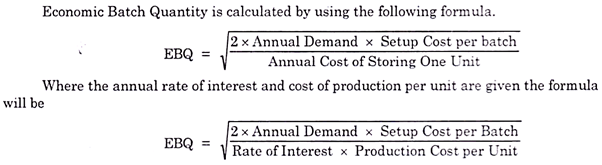### Batch Costing – Meaning and Definition

Batch Costing is used where the articles are produced in definite batches and held in stock either for assembly of components to produce finished product or for sales to customers severally or for both. For instance, in manufacturing component parts for final assembly of radio sets, watches, etc., Batch Costing can conveniently and gainfully be used. A Ready-made Garments Factory may make shirts in suitable batches according to size and keep in stock for sale on demand.

In this case, there is no meaning in keeping cost records for each shirt made as the cost of maintaining may exceed the benefit. Therefore, the cost records are kept and maintained in such a way that it will facilitate the ascertainment of cost of a batch (comprising of 100, 1000, 1500, etc., shirts) of a particular type of shirt.

To compute the cost of each shirt, the total cost of the batch is divided by the number of shirts in that batch. Therefore, a batch for which costs are ascertained is the cost unit in Batch Costing.

This Costing Method is primarily used in Biscuit Manufacture, Garments Manufacture, Manufacture of Spare Parts and Components, Manufacture of Shoes, Toys, Cars, Radios, Watches, etc.

ADVERTISEMENTS:

The procedure for ascertaining the cost of the batch is similar to that of Job Costing. As in the case of Job Costing, a number is allotted to each batch of production and accordingly, costs are accumulated. Material requisitions priced appropriately by the Costing Department are allocated directly to the batch concerned.

In the same way, direct labour costs attributable to each batch are ascertained and charged on the basis of the time sheets or job/batch tickets. Further, overhead expenses are absorbed on some suitable bases depending upon the policy of the company about the absorption of overhead expenses.

Batch costing is a modification or variation of job costing. Job costing is employed to deal with the costing of jobs undertaken as per specific orders of customers to suit their particular requirements. Batch costing is more suitable when articles are produced in definite batches and held in stock for future sale or stored as components for assembling a finished product.

According to I.C.M. A., London, “Batch Costing is that form of specific order costing which applies where similar articles are manufactured in batches either for sale-or use within the company”. A ‘Batch’ according to I.C.M. A., London is “a cost unit which consists of a group of similar articles which maintain its identity throughout one or more stages of production”.

ADVERTISEMENTS:

In watch making, Radio and T.V. assembling Batch Costing is extensively used to compute the cost of individual components. Similarly, in the manufacturing of toys, shoes, etc., also batch costing is employed to ascertain cost per finished unit of the product.

A ‘Batch’ is the ‘unit of cost’ in Batch costing. A separate ‘Batch order number’ is issued for each batch. Material requisitions and time sheets or job tickets’ provide the material and labour costs for the batch. Overheads are absorbed on some appropriate basis. A ‘Batch cost sheet’ is prepared to ascertain the total cost of a Batch and Cost per unit in the Batch. The Batch cost sheet is similar to job cost sheet.

### Batch Costing – Nature and Uses

Batch costing is a modified form of job costing. While job costing is concerned with costing of jobs that are executed against specific orders of the customers, batch costing is used where articles are manufactured in definite batches. The articles are usually kept in stock for selling to customers on demand.

The term batch refers to the ‘lot’ in which the articles are to be manufactured. Whenever a particular product is required, one unit of such product is not produced but a lot of ‘say’ 500 or 1,000 units of such product is produced. It is therefore also known as “Lot Costing”.

This method of costing is used in case of pharmaceutical or drug industries, ready-made garment factories, industries manufacturing component parts of radios, television sets, watches etc.The costing procedure for batch costing is similar to that under job costing except with the difference that a batch becomes the cost unit instead of a job.

Separate job cost sheets are maintained for each batch of products. Each batch is allotted a number. Material requisitions are prepared batch wise, the direct labour is engaged batch wise and the overheads are also recovered batch wise. Cost per unit is ascertained by dividing the total cost of a batch by number of items produced in that batch. Ordinary principles of inventory control are used.

ADVERTISEMENTS:

Production orders are issued only when the stock of finished goods reaches the ordering level. In case the batches are repetitive, the costing work is much simplified.

### Batch Costing – Top Features

1. The batch is the cost unit.

2. The batch cost sheet is prepared in the similar manner as it is done in case of job costing. It shows essentially the same information in respect of the batch that job cost sheet shows in respect of a job.

3. Economic batch quantity is calculated after considering set up cost, carrying cost and annual demand.

4. Batch Account is opened for each batch. All direct materials, direct labour and production overheads are debited to the Batch Account. After completion, batch cost is transferred to cost of sales.

Some of the other features are:

ADVERTISEMENTS:

i. Costs are collected batch-wise – A batch number is allocated to each batch and costs are accumulated for each batch.

ii. Products of identical nature – Items which are produced in a batch are identical or similar in nature.

iii. Convenient group – It is possible to classify items into convenient groups for the purpose of costing.

### Industries Where Batch Costing is Applied

A batch may be defined as a group of specified number of similar products. Under this method, a batch is treated as one job and the cost of this complete batch is ascertained. Batch costing is generally employed in industries engaged in biscuit manufacture, toy making, spare parts manufacture, canned products, ready-made garments, spare parts of complex products like motor cars, scooters, radios, watches etc.

This method is generally applied in those industries where the following features are present:

(i) The production is carried on in batches and each batch consists of a specified number of similar products.

ADVERTISEMENTS:

(ii) The production is done for own stock to be sold to customers.

(iii) The total cost of the batch is divided by the total number of units in the batch to find out the cost per unit.

(iv) Each batch is assigned a specified number or code which is mentioned on the products.

### 4 Essentials of Batch Costing

1. This method is applied where small parts of a considerable number are manufactured. (E.g. Machinery tools, machine tools etc.)

2. Complete production is divided into economic groups and appropriate costing methods are applied to each group.

3. The merits of the method are that the unit cost of each group is determined so that the total production cost is broken down into its constituent parts and profit and loss of each group is known separately.

ADVERTISEMENTS:

4. No special costing principles are involved, each group constituting a separate unit divided into subunits of each component produced.

### Economic Batch Quantity – Introduction, Equation, Illustration, Solution and Determination (with Formula)

Spare parts and components are produced one after another with the help of the same production facilities. But machines and tools are to be reset and adjusted each time when production is changed from one component to another. In this process machine operator and his assistant has to spend time and incur some expenditures for resetting the machines and tools.

So machines will be reset only when a minimum number of units are to be produced and also maximum number of units to be produced in a batch will also be fixed. Production of large quantities in a batch will be uneconomical since goods produced are to be stored for long before sale and more capital is locked up.

Setting up production for small quantities will also be uneconomical since set-up costs are to be borne by few units. So a quantity is to be fixed which is neither big nor small, that quantity will bring economy in production cost. That quantity to be produced in a batch is called Economic Batch Quantity (EBQ) or Economic Lot Size. Economic Batch Quantity can be calculated in three ways. One is trial-and-error method. Other way is using an equation which is similar to EOQ.

The equation is as follows: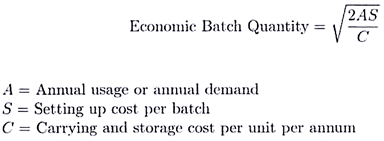Third method of calculation of Economic Batch Quantity is presenting the relevant information in a graph. The relevant informations are inventory holding cost, setting up cost and annual demand for the component.

Problem:

Annual demand for a component is 30,000 units. Cost of set-up per batch is Rs.600. Inventory carrying cost per unit per annum is Rs.1.

(a) Calculate the total cost assuming batch size of 4,000 units, 5,000 units, 6,000 units, 7,000 units, 8,000 units, 9,000 units and 10,000 units. Also find the economic batch quantity.

(b) Using mathematical formula calculate economic batch quantity.

Solution: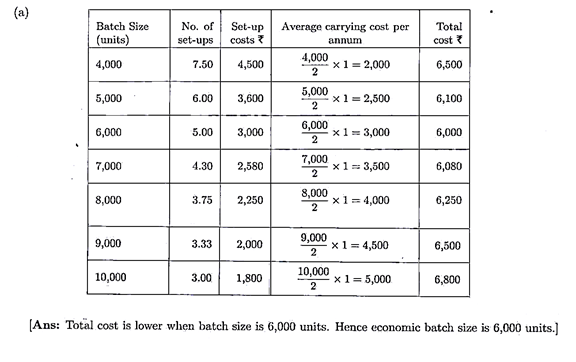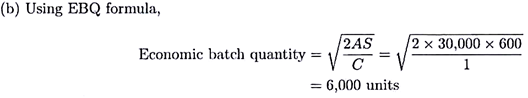Determination of Economic Batch Quantity:

One of the important aspects which needs the attention and decision of the concerned authority of industries where Batch Costing is employed is the determination of Economic Batch Quantity (EBQ). That means, the company has to decide about the size of each batch of production.

An appropriate decision about the size of each batch shall be taken after considering the relevant costs as the companies aim at minimization of costs. The costs which shall are considered are ‘set up costs’ and ‘carrying costs’.

1. Production of each batch of output necessitates the setting up of machines and tools. Hence, manufacture of a batch of output gives rise to incurrence of machine set up costs at the commencement of production, and machine disassembly costs on completion of the production of a batch.

i. These costs are incurred with each batch of production and they are, usually, independent of the size of the batch. That means, the set up costs of a batch remain constant irrespective of the number of units in the batch.

ii. Consequently, set up costs per unit of output can be reduced by increasing the size of the batch.

iii. Most importantly, the total annual set up costs increase with the decrease in size and vice-versa. Because, the decrease in size requires the increase in number of batches.

The following hypothetical illustration clarifies this point. Assume that the annual requirement of a component is 5,000 units and the set up cost per batch is Rs.20,000.

###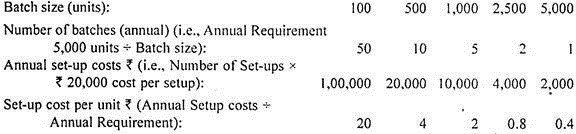From the above hypothetical illustration, it is obvious that the incidence of set-up costs (on each unit) can be lowered by increasing the batch size. Most important thing is that the annual set up costs can be lowered by maximizing the batch size which reduces the number of batches of production per annum. With a view to minimize the set up cost per unit, it is necessary, therefore, to maximize the size of the batch.

2. On the other hand, increase in the number of units in a batch (i.e., producing more units in a batch) results in accumulation of inventory over a period of time and therefore, results in higher carrying costs. Interest on capital locked up in unsold stock, cost of loss of stock in warehouse, etc., are examples to carrying costs. These costs increase with the increase in the number of units of unsold stock carried from one period of time to another.

Therefore, unsold stock shall be minimized to minimize the carrying costs. Hence, the batch size shall be lowered. A few figures presented below clarify these points. Assume, annual interest rate = 12% and unit manufacturing cost = Rs. 75.

###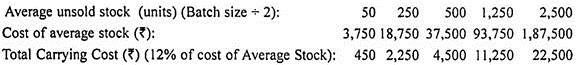From the analysis made hitherto, it is obvious that to minimize the annual total set up cost, the batch size shall be increased and to minimize the total carrying cost, the batch size shall be lowered. It is, therefore, necessary to strike a right balance between these two categories of costs to minimize the aggregate of these costs. The batch size which involves minimum costs for set up and carrying is the Economic Batch Quantity. This is similar to Economic Order Quantity.

As in the case of Economic Order Quantity, Economic Batch Quantity can be computed either by using Equational Approach or Graphical Approach.

A simple and widely used formula for computing Economic Batch Quantity is presented below:

###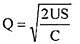Where,

Q = Economic Batch Quantity

U = Total number of units to be produced in a year

S = Set-up cost per batch

C = Carrying cost per unit of output per year. ‘C’ (viz., Carrying Cost per unit per year) can also be computed by multiplying the unit manufacturing cost by the annual interest rate.

### Batch Cost Sheet

For the purpose of costing, each batch or group of identical products is allotted a serial number (known as Batch Number) just like a job number. The presentation of the various items of cost is made in the form of a statement known as ‘Batch Cost Sheet.’

The Batch Cost Sheet includes the Cost of Direct Material, Cost of Direct Labour and Direct Expenses which can be directly identified with a particular batch. The share of overhead chargeable to the batch (calculated on some equitable basis) is also included in the statement. The total cost of a batch as shown by the Batch Cost Sheet divided by the total number of products in the batch will give us the cost per product in the batch.

Having determined the economic batch quantity, the batch cost sheet can be prepared. The batch cost sheet will show the setting up cost as well as production cost under distinct headings. Depending on the availability of data, it may also show the selling price and profit.

### Difference between Batch Costing and Job Costing

Batch costing is a form of job costing in which a batch of identical products is taken as the cost unit. A convenient batch of production is treated as a job. Then the batch cost is used to determine the unit cost of articles. It is a modification of job costing. Batch costing is used when the manufacturing consists of repetitive production.

Job costing is employed when jobs are executed for different customers according to their specifications. Each job is treated as a separate entity for the purpose of costing. Costs are collected and accumulated according to jobs.